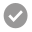Revista Internacional de Métodos Numéricos para Cálculo y Diseño en Ingeniería

##A stabilized meshless method for the solution of the lagrangian equations of newtonian fluids

Abstract

In this article we present numerical methods for the approximation of incompressible flows. We have addressed three problems: the stationary Stokes’ problem, the transient Stokes’ problem, and the general motion of newtonian fluids. In the three cases a discretization [...]

•123
Revista Internacional de Métodos Numéricos para Cálculo y Diseño en Ingeniería

##Stability and dispersion of the problem of seismic wave propagation in 2-D using the generalized finite difference method

Abstract

This paper shows the solution to the problem of seismic wave propagation in 2-D using generalized finite difference (GFD) explicit schemes. Regular and irregular meshes can be used with this method. As we are using an explicit method, it is necessary to obtain the stability condition [...]
•57
Papers Repository of the International Centre for Numerical Methods in Engineering (CIMNE)

##Fluid-structure interaction using the Particle Finite Element Method

Abstract

In the present work a new approach to solve fluid-structure interaction problems is described. Both, the equations of motion for fluids and for solids have been approximated using a material (lagrangian) formulation. To approximate the partial differential equations representing [...]

•50
Papers Repository of the International Centre for Numerical Methods in Engineering (CIMNE)

##The meshless finite element method

Abstract

A meshless method is presented which has the advantages of the good meshless methods concerning the ease of introduction of node connectivity in a bounded time of order [...]

•14
Papers Repository of the International Centre for Numerical Methods in Engineering (CIMNE)

##Simple modifications for stabilization of the finite point method

Abstract

A stabilized version of the finite point method (FPM) is presented. A source of instability due to the evaluation of the base function using a least square [...]

•14
Papers Repository of the International Centre for Numerical Methods in Engineering (CIMNE)

##A finite point method for elasticity problems

Abstract

The basis of the finite point method (FPM) for the fully meshless solution of elasticity problems in structural mechanics[...]
•27
Papers Repository of the International Centre for Numerical Methods in Engineering (CIMNE)

##A Lagrangian meshless finite element method applied to fluid–structure interaction problems

Abstract

A method is presented for the solution of the incompressible fluid flow equations using a Lagrangian formulation. The interpolation functions are [...]

•22
Revista Internacional de Métodos Numéricos para Cálculo y Diseño en Ingeniería

##Analysis of MFS-DRM formulations for nonhomogeneous diffusion problems

Abstract

This paper presents tests and applications related to the Method of the Fundamental Solutions (MFS) for nonhomogeneous diffusion equations. The dual reciprocity method was applied to approximate the particular solution and different MSF were applied to solve the homogeneous equation. [...]

•123
•5/5
Papers Repository of the International Centre for Numerical Methods in Engineering (CIMNE)

##A finite point method for adaptive three‐dimensional compressible flow calculations

Abstract

The finite point method (FPM) is a meshless technique, which is based on both, a weighted least‐squares numerical approximation on local clouds of points and a collocation technique which [...]

•23